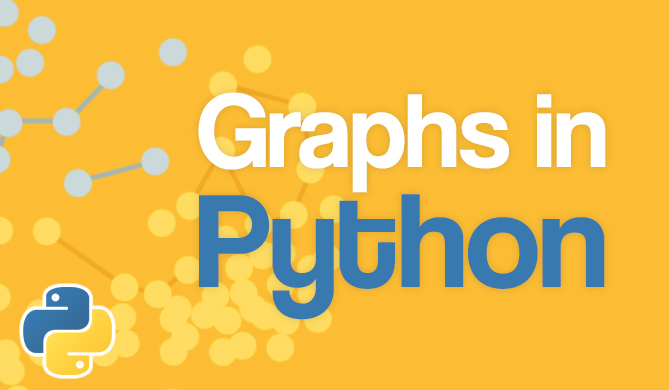JavaScript: Check if Object is Array

# JavaScript: Check if Object is Array## Introduction

Working with arrays in JavaScript is a common activity. Sometimes we get a variable in JavaScript that we need to be an array, but we aren't sure that it is.

Non-primitive data types in JavaScripts are all objects (functions have their own type, but they too are objects). As a result, it's not sufficient to use the typeof operator that's commonly used to determine a data type:

let result = { subject: 'Science', marks: 97 };
let numbers = [1, 2, 3, 4, 5];

console.log(typeof result); // Object
console.log(typeof numbers); // Object


In this article, we'll take a look at how to check if a given variable or value is an array or not, in JavaScript.

## Using the Array.isArray() Method

As the name suggests, this method can be used to identify whether the given argument is an array or not. It returns a boolean (true/false) value with the result.

For example, with the following variables the Array.isArray() method correctly determines if they are one or not:

let result = { subject: "Science", marks: 97 }; // Object
let numbers = [1, 2, 3, 4, 5]; // Array
let name = "Mark"; // String
let names = new Array("Jill", "Jane", "Jacqueline");

console.log(Array.isArray(result)); // false
console.log(Array.isArray(numbers)); // true
console.log(Array.isArray(name)); // false
console.log(Array.isArray(names)); // true


## Using an Object's constructor Property

Every object has a constructor property (except objects created with Object.create(null), an unlikely occurrence). We can compare the constructor property directly with JavaScript's constructor functions. Therefore, if we compare it with the array constructor function, we'll know if it's an array.Note: A constructor function is a function that initializes an object. If you've created an object with the new keyword, you did so with a constructor function. For example, in let myArray = new Array(1, 2) the constructor function used is Array().

You can use the constructor property to determine if a variable is an array:

Free eBook: Git Essentials

Check out our hands-on, practical guide to learning Git, with best-practices, industry-accepted standards, and included cheat sheet. Stop Googling Git commands and actually learn it!

let result = { subject: "Science", marks: 97 };
let numbers = [1, 2, 3, 4, 5];
let name = "Mark";
let names = new Array("Jill", "Jane", "Jacqueline");

console.log(result.constructor === Array); // false
console.log(numbers.constructor === Array); // true
console.log(name.constructor === Array); // false
console.log(names.constructor === Array); // true


## Using the instanceof Operator

The instanceof operator checks if a constructor function is found in the prototype chain of an object. If you're less familiar with JavaScript's prototypal inheritance, the operator checks if an object was created by a class, and if not, checks if the object was derived from that class.

Like the typeof operator, it returns a boolean value. To determine if a variable is an array, we can use instanceof like this:

let result = { subject: "Science", marks: 97 };
let numbers = [1, 2, 3, 4, 5];
let name = "Mark";
let names = new Array("Jill", "Jane", "Jacqueline");

console.log(result instanceof Array); // false
console.log(numbers instanceof Array); // true
console.log(name instanceof Array); // false
console.log(names instanceof Array); // true


## Using the Object.prototype.call() Method

All objects in JavaScript inherit properties from the main prototype object, aptly named Object.prototype. A toString() method exists in Object.prototype, which is why every object has a toString() method of their own. The toString() method of Object.prototype displays the type of an object.

The call() method of objects executes a function but changes the value of this to the object passed in its arguments i.e. it allows an object to use a method from another object.

Therefore, we can use Object.prototype.toString() to print the type and then use call() so it's done for another object. We then compare that string value to determine if it's an array:

let result = { subject: "Science", marks: 97 };
let numbers = [1, 2, 3, 4, 5];
let name = "Mark";
let names = new Array("Jill", "Jane", "Jacqueline");

console.log(Object.prototype.toString.call(result)); // [object Object]
console.log(Object.prototype.toString.call(numbers)); // [object Array]
console.log(Object.prototype.toString.call(name)); // [object String]
console.log(Object.prototype.toString.call(names)); // [object Array]

console.log(Object.prototype.toString.call(result) === "[object Array]"); // false
console.log(Object.prototype.toString.call(numbers) === "[object Array]"); // true
console.log(Object.prototype.toString.call(name) === "[object Array]"); // false
console.log(Object.prototype.toString.call(names) === "[object Array]"); // true


It's unlikely that you'll use this method, but it never hurts to know more about JavaScript objects!

## Conclusion

In this article, we looked at a few ways in JavaScript to determine if an object is an array. The easiest method is the Array.isArray() method that will most likely be used in production.

However, we can always leverage the instanceof operator and other object properties to determine if it's an array.

Last Updated: March 9th, 2023

Get tutorials, guides, and dev jobs in your inbox.Free
Course

### Graphs in Python - Theory and Implementation

# python# data structures# algorithms# computer science

Graphs are an extremely versatile data structure. More so than most people realize! Graphs can be used to model practically anything, given their nature of...DetailsProject

### React State Management with Redux and Redux-Toolkit

# javascript# React

Coordinating state and keeping components in sync can be tricky. If components rely on the same data but do not communicate with each other when...Details